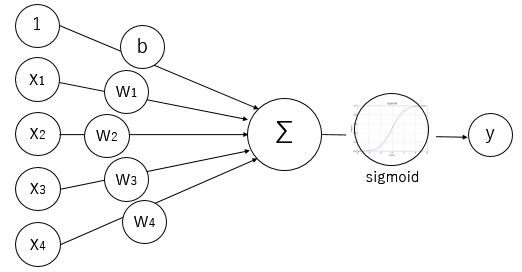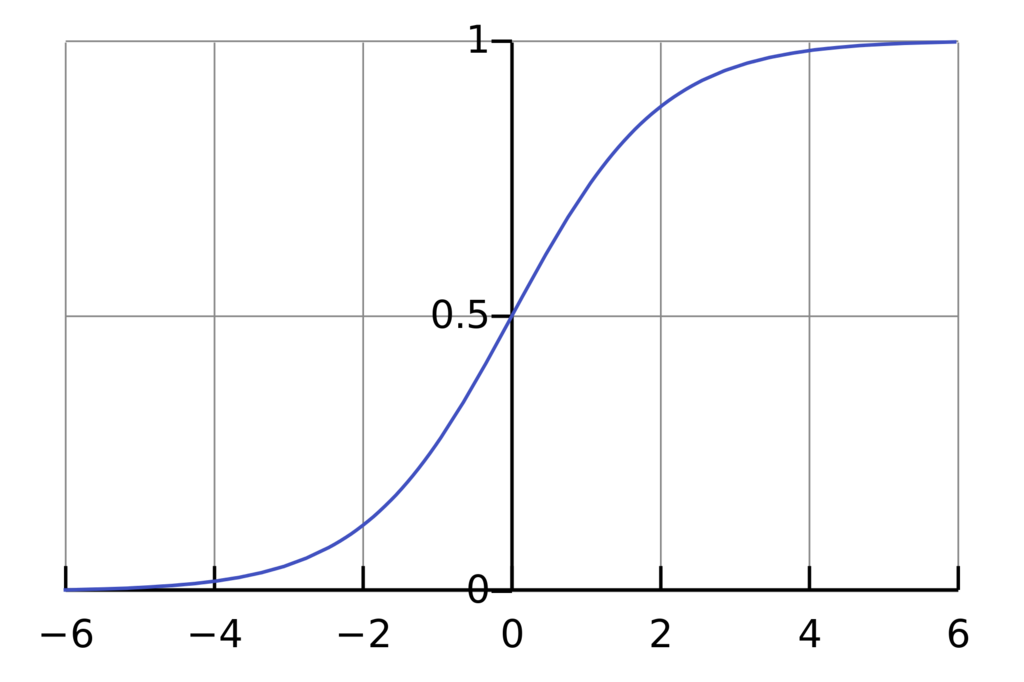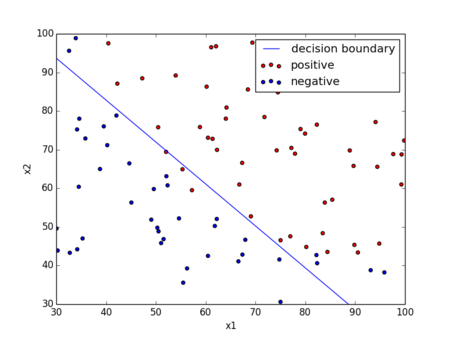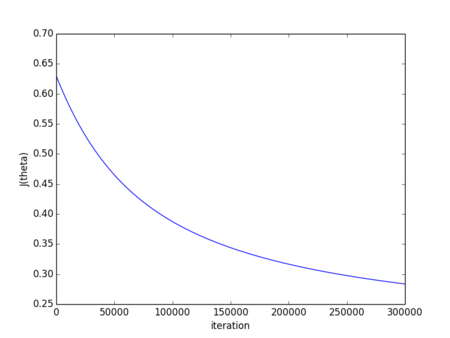Logistic Regression.

I'm gonna note down here about Logistic Regression because I learned it again at Cousera's Machine Learning course. In machine learning, Logistic Regression is often used for classification. It measures the relationship between the categorical dependent variable and one or more independent variables by estimating probabilities using a logistic function, which is the cumulative logistic distribution and it called binomial distribution. In case of classification (ex, Tumor size predicts malignant potential. y=1 is Yes, y=0 is No.), y = 0 or 1, if you use linear regression,can be >1 or < 0. But this is bad because of expecting y = 0 or 1. So it is a good way to use Logistic Regression because it is actually a classification algorithm that we apply to settings where the label y is discrete value, when it's either 0 or 1.

Hypothesis Representation of Logistic Regression

Logistic Regression Model is below:WantModelSigmoid functionAssigning sigmoid function into model, we can get Logistic Regression Model below:

ModelUsing this model, you can draw below graph and see it asymptotes at one and zero.Logistic regression - Wikipedia, the free encyclopedia

For interpretation of hypothesis output, this model estimates probability that y=1 on input x. For example of tumor size predicts malignant potential, x is given below:It may(means probability y = 1), doctor tell patient that 70% change of tumor being malignant.means "probability that y=1, given x, parameterized by θ." and this y is 0 or 1.

Decision Boundary of Logistic Regression

Logistic regression supposes:
prediction "y=1" if0.5

prediction "y=0" if< 0.5

Seeing above graph, x bar means z and z0 be y bar0.5, z < 0 be y bar < 0.5. this means.
And we can draw linear with each θ values. For example, it given below:
ifand θ=[-3, 1, 1], you can predict "y=1" if.
It can be changedandwhich make it decide Decision Boundary and means 0.5.

Cost Function of Logistic Regression

How to choose parameters θ(how to fit data)? In Logistic regression, Cost function is below:
If y = 1,If y = 0,Overall cost function j of θ will be convex and local optima free contrast with Linear regression because it will be log function's graph. If it missed for prediction, you have to pay more cost.
You can capture intuition that if(predict), but y=1 which means that we will penalize learning algorithm by a very large cost.This is the Cross-entropy error function which is an idea in statistics for how to efficiently find parameter's data for different models. This cost function can be derived from statistics using the principle of maximum likelihood estimation. Finding min J(θ) as fitting parameters θ to make a prediction given new x. You have to repeat computing a below gradient descent with simultaneously updating all θ :You will notice that this algorithm looks identical to linear regression. But hypothesis is difference between Logistic regression and Linear regression below:

Logistic regression :Linear regression :#coding: utf-8

import numpy as np
import matplotlib.pyplot as plt

def plotData(X, y):
positive = [i for i in range(len(y)) if y[i] == 1]
negative = [i for i in range(len(y)) if y[i] == 0]

plt.scatter(X[positive, 0], X[positive, 1], c='red', marker='o', label="positive")
plt.scatter(X[negative, 0], X[negative, 1], c='blue', marker='o', label="negative")

def sigmoid(z):
return 1.0 / (1 + np.exp(-z))

def safe_log(x, minval=0.0000000001):
return np.log(x.clip(min=minval))

def computeCost(X, y, theta):
h = sigmoid(np.dot(X, theta))
J = (1.0 / m) * np.sum(-y * safe_log(h) - (1 - y) * safe_log(1 - h))
return J

def gradientDescent(X, y, theta, alpha, iterations):
m = len(y)      # length of training data
J_history = []  # cost of each update
for iter in range(iterations):
h = sigmoid(np.dot(X, theta))
theta = theta - alpha * (1.0 / m) * np.dot(X.T, h - y)
cost = computeCost(X, y, theta)
print iter, cost
J_history.append(cost)
return theta, J_history

def main():
data = np.genfromtxt("ex2data1.txt", delimiter=",")
X = data[:, (0, 1)]
y = data[:, 2]
m = len(y)

plt.figure(1)
plotData(X, y)

X = X.reshape((m, 2))
X = np.hstack((np.ones((m, 1)), X))

# initialize parameters to 0
theta = np.zeros(3)
iterations = 300000
alpha = 0.001

# compute cost as initialize
initialCost = computeCost(X, y, theta)
print "initial cost:", initialCost

# estimate parameters using gradient descent
theta, J_history = gradientDescent(X, y, theta, alpha, iterations)
print "theta:", theta
print "final cost:", J_history[-1]

plt.figure(2)
plt.plot(J_history)
plt.xlabel("iteration")
plt.ylabel("J(theta)")

plt.figure(1)
xmin, xmax = min(X[:,1]), max(X[:,1])
xs = np.linspace(xmin, xmax, 100)
ys = [- (theta / theta) - (theta / theta) * x for x in xs]
plt.plot(xs, ys, 'b-', label="decision boundary")
plt.xlabel("x1")
plt.ylabel("x2")
plt.xlim((30, 100))
plt.ylim((30, 100))
plt.legend()
plt.show()

if __name__ == "__main__":
main()


This result is below:

initial cost: 0.69314718056
theta: [-9.25573205  0.07960975  0.07329322]
final cost: 0.283686931959